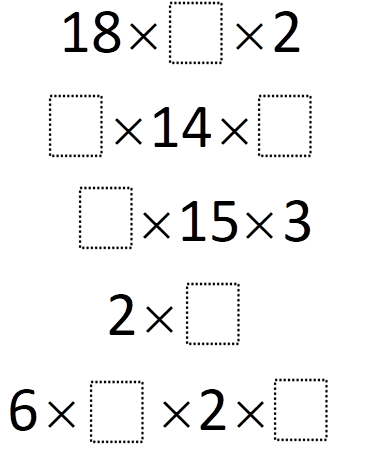# Perfect Squares

Directions: Using the digits 1- 9, at most one time each, to fill in the boxes to make each expression evaluate to a perfect square number.

Extension/Challenge: What is the largest/smallest square number you can make? How many different perfect square numbers could be made?### Hint

You may find it helpful to factor each number in the expression into their prime factors first.

Number of Unique Solutions: 12

#1:
18 x 1 x 2 = 36
2 x 14 x 7 = 196
5 x 15 x 3 = 225
2 x 8 = 16
6 x 3 x 2 x 9 = 324

#2:
18 x 1 x 2 = 36
7 x 14 x 2 = 196
5 x 15 x 3 = 225
2 x 8 = 16
6 x 3 x 2 x 4 = 144

#3:
18 x 1 x 2 = 36
7 x 14 x 8 = 784
5 x 15 x 3 = 225
2 x 2 = 4
6 x 3 x 2 x 9 = 324

#4:
18 x 1 x 2 = 36
8 x 14 x 7 = 784
5 x 15 x 3 = 225
2 x 2 = 4
6 x 4 x 2 x 3 = 144

#5:
18 x 4 x 2 = 144
7 x 14 x 2 = 196
5 x 15 x 3 = 225
2 x 8 = 16
6 x 3 x 2 x 1 = 36

#6:
18 x 4 x 2 = 144
7 x 14 x 2 = 196
5 x 15 x 3 = 225
2 x 8 = 16
6 x 9 x 2 x 3 = 324

#7:
18 x 4 x 2 = 144
7 x 14 x 8 = 784
5 x 15 x 3 = 225
2 x 2 = 4
6 x 3 x 2 x 1 = 36

#8:
18 x 4 x 2 = 144
8 x 14 x 7 = 784
5 x 15 x 3 = 225
2 x 2 = 4
6 x 9 x 2 x 3 = 324

#9:
18 x 9 x 2 = 324
7 x 14 x 2 = 196
5 x 15 x 3 = 225
2 x 8 = 16
6 x 3 x 2 x 1 = 36

#10:
18 x 9 x 2 = 324
7 x 14 x 2 = 196
5 x 15 x 3 = 225
2 x 8 = 16
6 x 4 x 2 x 3 = 144

#11:
18 x 9 x 2 = 324
7 x 14 x 8 = 784
5 x 15 x 3 = 225
2 x 2 = 4
6 x 3 x 2 x 1 = 36

#12:
18 x 9 x 2 = 324
7 x 14 x 8 = 784
5 x 15 x 3 = 225
2 x 2 = 4
6 x 3 x 2 x 4 = 144

Source: Erick Lee

## Square Root Expression 2

Directions: Using the digits 0 to 9 at most one time each, place a digit …

1.18 x 4 x 2 = 144
2 x 14 x 7 = 196
5 x 15 x 3 = 225
2 x 8 = 16
6 x 1 x 2 x 3 = 36

I used factors of the given numbers and then matched them up to make sure that there were two of each which would make them perfect squares.

••2.